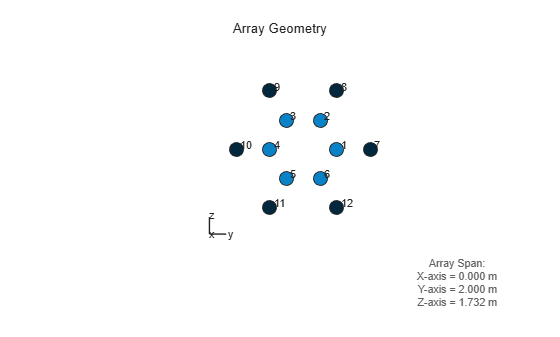# getTaper

System object: phased.HeterogeneousConformalArray
Package: phased

Array element tapers

## Syntax

```wts = getTaper(h) ```

## Description

`wts = getTaper(h)` returns the tapers applied to each element of a conformal array, `h`. Tapers are often referred to as weights.

## Input Arguments

expand all

Conformal array specified as a `phased.HeterogeneousConformalArray` System object™.

## Output Arguments

expand all

Array element tapers returned as an N-by-1, complex-valued vector, where N is the number of elements in the array.

## Examples

expand all

Create a 12-element, 2-ring tapered disk array where the outer ring is more heavily tapered than the inner ring.

```antenna1 = phased.ShortDipoleAntennaElement('FrequencyRange',[100e6 1e9], ... 'AxisDirection','Z'); antenna2 = phased.ShortDipoleAntennaElement('FrequencyRange',[100e6 1e9], ... 'AxisDirection','Y'); elemAngles = ([0:5]*360/6); elemPosInner = 0.5*[zeros(size(elemAngles));cosd(elemAngles); ... sind(elemAngles)]; elemPosOuter = [zeros(size(elemAngles));cosd(elemAngles); ... sind(elemAngles)]; elemNorms = repmat([0;0],1,12); taper = [ones(size(elemAngles)),0.3*ones(size(elemAngles))]; array = phased.HeterogeneousConformalArray('ElementSet',{antenna1,antenna2}, ... 'ElementIndices',[1 1 1 1 1 1 2 2 2 2 2 2], ... 'ElementPosition',[elemPosInner,elemPosOuter],'ElementNormal',elemNorms, ... 'Taper',taper); w = getTaper(array)```
```w = 12×1 1.0000 1.0000 1.0000 1.0000 1.0000 1.0000 0.3000 0.3000 0.3000 0.3000 ⋮ ```

Draw the array showing taper colors.

`viewArray(array,'ShowTaper',true,'ShowIndex','all')`